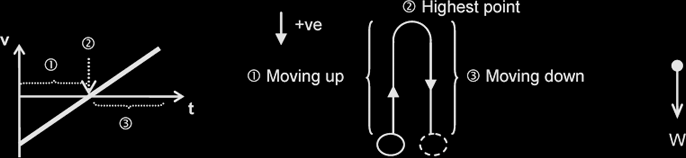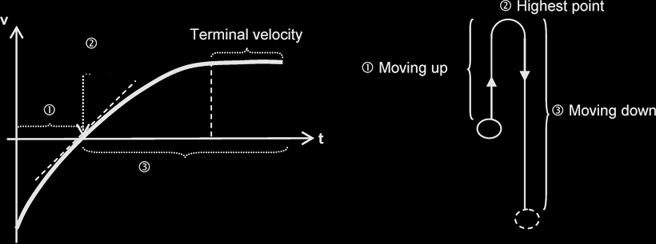# Kinematics

#### Displacement, speed, velocity and acceleration:

Distance: Total length covered irrespective of the direction of motion.

Displacement: Distance moved in a certain direction.

Speed: Distance travelled per unit time.

Velocity: is defined as the rate of change of displacement, or, displacement per unit time
{NOT: displacement over time, nor, displacement per second, nor, rate of change of displacement per unit time}

Acceleration: is defined as the rate of change of velocity.

#### Using graphs to find displacement, velocity and acceleration:

• The area under a velocity-time graph is the change in displacement.
• The gradient of a displacement-time graph is the {instantaneous} velocity.
• The gradient of a velocity-time graph is the acceleration.

#### The 'SUVAT' Equations of Motion

The most important word for this chapter is SUVAT, which stands for:

• S (displacement),
• U (initial velocity),
• V (final velocity),
• A (acceleration) and
• T (time)

of a particle that is in motion.

Below is a list of the equations you MUST memorise, even if they are in the formula book, memorise them anyway, to ensure you can implement them quickly.

 1 v = u +at derived from definition of acceleration: a = (v – u) / t 2 s = ½ (u + v) t derived from the area under the v-t graph 3 v2 = u2 + 2as derived from equations (1) and (2) 4 s = ut + ½at2 derived from equations (1) and (2)

These equations apply only if the motion takes place along a straight line and the acceleration is constant; {hence, for eg., air resistance must be negligible.}

### Motion of bodies falling in a uniform gravitational field with air resistance:

Consider a body moving in a uniform gravitational field under 2 different conditions:

#### Without Air Resistance:Assuming negligible air resistance, whether the body is moving up, or at the highest point or moving down, the weight of the body, W, is the only force acting on it, causing it to experience a constant acceleration. Thus, the gradient of the v-t graph is constant throughout its rise and fall. The body is said to undergo free fall.

#### With Air Resistance:If air resistance is NOT negligible and if it is projected upwards with the same initial velocity, as the body moves upwards, both air resistance and weight act downwards. Thus its speed will decrease at a rate greater than 9.81 ms-2 . This causes the time taken to reach its maximum height reached to be lower than in the case with no air resistance. The max height reached is also reduced.

At the highest point, the body is momentarily at rest; air resistance becomes zero and hence the only force acting on it is the weight. The acceleration is thus 9.81 ms-2 at this point.

As a body falls, air resistance opposes its weight. The downward acceleration is thus less than 9.81 ms-2. As air resistance increases with speed, it eventually equals its weight (but in opposite direction). From then there will be no resultant force acting on the body and it will fall with a constant speed, called the terminal velocity.

#### Equations for the horizontal and vertical motion:

x direction (horizontal – axis) y direction (vertical – axis)
s (displacement) sx = ux t
sx = ux t + ½ax t2
sy = uy t + ½ ay t2
(Note: If projectile ends at same level as the start, then sy = 0)
u (initial velocity) ux uy
v (final velocity) vx = ux + axt
(Note: At max height, vx = 0)
vy = uy + at
vy2 = uy2 + 2asy
a (acceleration) ax
(Note: Exists when a force in x direction present)
ay
(Note: If object is falling, then ay = -g)
t (time) t t

Parabolic Motion: tan θ = vy / vx
θ: direction of tangential velocity {NOT: tan θ = sy / sx }